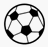Skill 3A
"Crossing Out" to solve subtraction problems
The procedure of "crossing-out" is very helpful for teaching subtraction to early elementary students.  Crossing-out shows students how numbers become smaller in subtraction while also giving them a strategy for easily solving subtraction problems.  It is very helpful with word problems as well, which will be covered in upcoming skills.

Look at the subtraction number sentence below 5 - 4, but do not tell me the answer right away.

What I want you to do is to tell me how many soccer balls you would cross-out to figure out the subtraction problem.  Then tell me how many soccer balls are left.

5 - 4 = ______